NEET  >  Case Based Questions Test: System of Particles & Rotational Motion

# Case Based Questions Test: System of Particles & Rotational Motion - NEET

Test Description

## 10 Questions MCQ Test Physics Class 11 - Case Based Questions Test: System of Particles & Rotational Motion

Case Based Questions Test: System of Particles & Rotational Motion for NEET 2023 is part of Physics Class 11 preparation. The Case Based Questions Test: System of Particles & Rotational Motion questions and answers have been prepared according to the NEET exam syllabus.The Case Based Questions Test: System of Particles & Rotational Motion MCQs are made for NEET 2023 Exam. Find important definitions, questions, notes, meanings, examples, exercises, MCQs and online tests for Case Based Questions Test: System of Particles & Rotational Motion below.
Solutions of Case Based Questions Test: System of Particles & Rotational Motion questions in English are available as part of our Physics Class 11 for NEET & Case Based Questions Test: System of Particles & Rotational Motion solutions in Hindi for Physics Class 11 course. Download more important topics, notes, lectures and mock test series for NEET Exam by signing up for free. Attempt Case Based Questions Test: System of Particles & Rotational Motion | 10 questions in 20 minutes | Mock test for NEET preparation | Free important questions MCQ to study Physics Class 11 for NEET Exam | Download free PDF with solutions
 1 Crore+ students have signed up on EduRev. Have you?
Case Based Questions Test: System of Particles & Rotational Motion - Question 1

### Position of centre of massIn a uniform gravitational field the centre of mass coincide with the centre of gravity. But these two points do not always coincide, however. For example, the Moon’s centre of mass is very close to its geometric centre (it is not exact because the Moon is not a perfect uniform sphere), but its centre of gravity is slightly displaced towards Earth because of the stronger gravitational force on the Moon’s near side facing the earth. If an object does not have a uniform weight distribution then the center of mass will be closer to where most of the weight is located. For example, the center of gravity for a hammer is located close to where the head connects to the handle. The center of mass can be located at an empty point in space, such as the center of a hollow ball. The center of gravity can even be completely outside of an object, such as for a donut or a curved banana.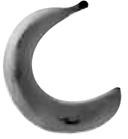Standing upright, an adult human’s centre of mass is located roughly at the center of their torso. The centre of mass rises a few inches when with rising arms.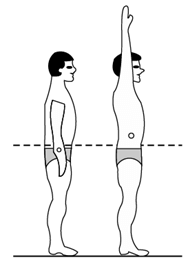The center of gravity can even be at a point outside the body, such as when bent over in an inverted-U pose.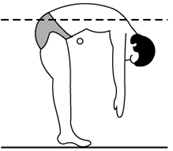An object is in balanced position if its center of gravity is above its base of support. For the two cylinders below, the left cylinder’s CG is above the base of support so the upward support force from the base is aligned with the downward force of gravity. For the cylinder on the right the CG is not above the base of support so these two forces cannot align and instead create a torque that rotates the object, tipping it over.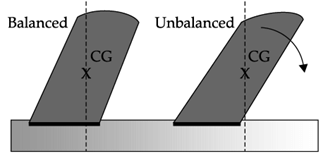Does the centre of mass does not coincide with the centre of gravity of a body?

Detailed Solution for Case Based Questions Test: System of Particles & Rotational Motion - Question 1
In a uniform gravitational field the centre of mass coincide with the centre of gravity.
Case Based Questions Test: System of Particles & Rotational Motion - Question 2

### Position of centre of massIn a uniform gravitational field the centre of mass coincide with the centre of gravity. But these two points do not always coincide, however. For example, the Moon’s centre of mass is very close to its geometric centre (it is not exact because the Moon is not a perfect uniform sphere), but its centre of gravity is slightly displaced towards Earth because of the stronger gravitational force on the Moon’s near side facing the earth. If an object does not have a uniform weight distribution then the center of mass will be closer to where most of the weight is located. For example, the center of gravity for a hammer is located close to where the head connects to the handle. The center of mass can be located at an empty point in space, such as the center of a hollow ball. The center of gravity can even be completely outside of an object, such as for a donut or a curved banana.Standing upright, an adult human’s centre of mass is located roughly at the center of their torso. The centre of mass rises a few inches when with rising arms.The center of gravity can even be at a point outside the body, such as when bent over in an inverted-U pose.An object is in balanced position if its center of gravity is above its base of support. For the two cylinders below, the left cylinder’s CG is above the base of support so the upward support force from the base is aligned with the downward force of gravity. For the cylinder on the right the CG is not above the base of support so these two forces cannot align and instead create a torque that rotates the object, tipping it over.Identical blocks are connected as shown. Where the center of mass is expected to be located?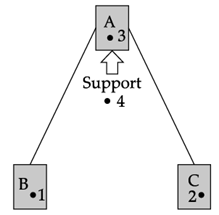Detailed Solution for Case Based Questions Test: System of Particles & Rotational Motion - Question 2
Since all the 3 blocks are identical, the centre of mass will be below the support but above the line joining the blocks B and C
Case Based Questions Test: System of Particles & Rotational Motion - Question 3

### Position of centre of massIn a uniform gravitational field the centre of mass coincide with the centre of gravity. But these two points do not always coincide, however. For example, the Moon’s centre of mass is very close to its geometric centre (it is not exact because the Moon is not a perfect uniform sphere), but its centre of gravity is slightly displaced towards Earth because of the stronger gravitational force on the Moon’s near side facing the earth. If an object does not have a uniform weight distribution then the center of mass will be closer to where most of the weight is located. For example, the center of gravity for a hammer is located close to where the head connects to the handle. The center of mass can be located at an empty point in space, such as the center of a hollow ball. The center of gravity can even be completely outside of an object, such as for a donut or a curved banana.Standing upright, an adult human’s centre of mass is located roughly at the center of their torso. The centre of mass rises a few inches when with rising arms.The center of gravity can even be at a point outside the body, such as when bent over in an inverted-U pose.An object is in balanced position if its center of gravity is above its base of support. For the two cylinders below, the left cylinder’s CG is above the base of support so the upward support force from the base is aligned with the downward force of gravity. For the cylinder on the right the CG is not above the base of support so these two forces cannot align and instead create a torque that rotates the object, tipping it over.Centre of gravity of Moon is slightly displaced towards Earth because

Detailed Solution for Case Based Questions Test: System of Particles & Rotational Motion - Question 3
The Moon’s centre of mass is very close to its geometric centre (it is not exact because the Moon is not a perfect uniform sphere), but its centre of gravity is slightly displaced towards Earth due to uneven gravitational field. The gravitational force is stronger on the Moon’s near side facing the earth.
Case Based Questions Test: System of Particles & Rotational Motion - Question 4

Position of centre of mass

In a uniform gravitational field the centre of mass coincide with the centre of gravity. But these two points do not always coincide, however. For example, the Moon’s centre of mass is very close to its geometric centre (it is not exact because the Moon is not a perfect uniform sphere), but its centre of gravity is slightly displaced towards Earth because of the stronger gravitational force on the Moon’s near side facing the earth. If an object does not have a uniform weight distribution then the center of mass will be closer to where most of the weight is located. For example, the center of gravity for a hammer is located close to where the head connects to the handle. The center of mass can be located at an empty point in space, such as the center of a hollow ball. The center of gravity can even be completely outside of an object, such as for a donut or a curved banana.Standing upright, an adult human’s centre of mass is located roughly at the center of their torso. The centre of mass rises a few inches when with rising arms.The center of gravity can even be at a point outside the body, such as when bent over in an inverted-U pose.An object is in balanced position if its center of gravity is above its base of support. For the two cylinders below, the left cylinder’s CG is above the base of support so the upward support force from the base is aligned with the downward force of gravity. For the cylinder on the right the CG is not above the base of support so these two forces cannot align and instead create a torque that rotates the object, tipping it over.Two similar blocks are shown below. The first block is top-heavy and the second block is bottom heavy. Where the centers of mass will be located?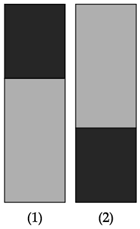Detailed Solution for Case Based Questions Test: System of Particles & Rotational Motion - Question 4
If an object does not have a uniform weight distribution then the center of mass will be closer to where most of the weight is located.
Case Based Questions Test: System of Particles & Rotational Motion - Question 5

Position of centre of mass

In a uniform gravitational field the centre of mass coincide with the centre of gravity. But these two points do not always coincide, however. For example, the Moon’s centre of mass is very close to its geometric centre (it is not exact because the Moon is not a perfect uniform sphere), but its centre of gravity is slightly displaced towards Earth because of the stronger gravitational force on the Moon’s near side facing the earth. If an object does not have a uniform weight distribution then the center of mass will be closer to where most of the weight is located. For example, the center of gravity for a hammer is located close to where the head connects to the handle. The center of mass can be located at an empty point in space, such as the center of a hollow ball. The center of gravity can even be completely outside of an object, such as for a donut or a curved banana.Standing upright, an adult human’s centre of mass is located roughly at the center of their torso. The centre of mass rises a few inches when with rising arms.The center of gravity can even be at a point outside the body, such as when bent over in an inverted-U pose.An object is in balanced position if its center of gravity is above its base of support. For the two cylinders below, the left cylinder’s CG is above the base of support so the upward support force from the base is aligned with the downward force of gravity. For the cylinder on the right the CG is not above the base of support so these two forces cannot align and instead create a torque that rotates the object, tipping it over.The leaning tower of Pisa does not fall since

Detailed Solution for Case Based Questions Test: System of Particles & Rotational Motion - Question 5
The Leaning Tower of Pisa does not fall because its center of mass has been carefully kept within its base. Today, despite the inclination of about 4° (reduced from over 5° ), a vertical line drawn from the center of mass still falls inside the base.
Case Based Questions Test: System of Particles & Rotational Motion - Question 6

Flywheel and sewing machine

There is a difference between inertia and moment of inertia of a body. Inertia depends on the mass of the body but the moment of inertia about an axis depends on the mass of the body and the distribution of its mass about the axis. In the following figure, the masses of the two wheels are exactly equal but in the wheel (A) the mass is uniformly distributed and in the wheel (B) most of the mass is situated at the rim. Both the wheels rotate about axis passing through the centre. It is noticed that while the two wheel are set in rotation and left, wheel (B) continues rotating for a longer time.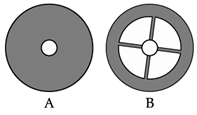This means that the moment of inertia of wheel (B) is greater than the wheel (B). Also greater is the part of the mass of the body away from the axis of rotation, greater the moment of inertia of the body about the axis. Such a wheel is known as flywheel. Consider a foot operated sewing machine. It has of two wheels – one big and the other small. The wheels are connected by a rope. The bigger wheel acts as flywheel. The rope transfers the motion from this flywheel to the smaller wheel. Smaller wheel works as a pulley and also as a small flywheel.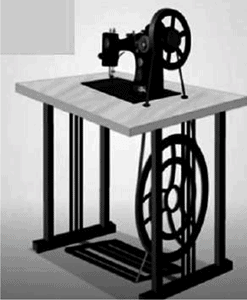We see even we stop supply of driving force to the bigger wheel it still continues to run for a short time because of its moment of inertia. So, flywheel acts as an energy reservoir by storing and supplying mechanical energy when required. The kinetic energy stored in a flywheel is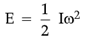(where I = moment of inertia and ω = angular velocity).

Which of the following statement is true?

Detailed Solution for Case Based Questions Test: System of Particles & Rotational Motion - Question 6
Inertia depends on the mass of the body but the moment of inertia about an axis depends on the mass of the body and the distribution of its mass about the axis. Moment of inertia = ∑mr2
Case Based Questions Test: System of Particles & Rotational Motion - Question 7

Flywheel and sewing machine

There is a difference between inertia and moment of inertia of a body. Inertia depends on the mass of the body but the moment of inertia about an axis depends on the mass of the body and the distribution of its mass about the axis. In the following figure, the masses of the two wheels are exactly equal but in the wheel (A) the mass is uniformly distributed and in the wheel (B) most of the mass is situated at the rim. Both the wheels rotate about axis passing through the centre. It is noticed that while the two wheel are set in rotation and left, wheel (B) continues rotating for a longer time.This means that the moment of inertia of wheel (B) is greater than the wheel (B). Also greater is the part of the mass of the body away from the axis of rotation, greater the moment of inertia of the body about the axis. Such a wheel is known as flywheel. Consider a foot operated sewing machine. It has of two wheels – one big and the other small. The wheels are connected by a rope. The bigger wheel acts as flywheel. The rope transfers the motion from this flywheel to the smaller wheel. Smaller wheel works as a pulley and also as a small flywheel.We see even we stop supply of driving force to the bigger wheel it still continues to run for a short time because of its moment of inertia. So, flywheel acts as an energy reservoir by storing and supplying mechanical energy when required. The kinetic energy stored in a flywheel is(where I = moment of inertia and ω = angular velocity).

How many flywheels are there in foot operated sewing machine ?

Detailed Solution for Case Based Questions Test: System of Particles & Rotational Motion - Question 7
In foot operated sewing machine there are two wheels – one big and the other small. The wheels are connected by a rope. The bigger wheel acts as flywheel. The rope transfers the motion from this flywheel to the smaller wheel. Smaller wheel works as a pulley and also as a small flywheel.
Case Based Questions Test: System of Particles & Rotational Motion - Question 8

Flywheel and sewing machine

There is a difference between inertia and moment of inertia of a body. Inertia depends on the mass of the body but the moment of inertia about an axis depends on the mass of the body and the distribution of its mass about the axis. In the following figure, the masses of the two wheels are exactly equal but in the wheel (A) the mass is uniformly distributed and in the wheel (B) most of the mass is situated at the rim. Both the wheels rotate about axis passing through the centre. It is noticed that while the two wheel are set in rotation and left, wheel (B) continues rotating for a longer time.This means that the moment of inertia of wheel (B) is greater than the wheel (B). Also greater is the part of the mass of the body away from the axis of rotation, greater the moment of inertia of the body about the axis. Such a wheel is known as flywheel. Consider a foot operated sewing machine. It has of two wheels – one big and the other small. The wheels are connected by a rope. The bigger wheel acts as flywheel. The rope transfers the motion from this flywheel to the smaller wheel. Smaller wheel works as a pulley and also as a small flywheel.We see even we stop supply of driving force to the bigger wheel it still continues to run for a short time because of its moment of inertia. So, flywheel acts as an energy reservoir by storing and supplying mechanical energy when required. The kinetic energy stored in a flywheel is(where I = moment of inertia and ω = angular velocity).

We see even we stop supply of driving force to the bigger wheel of foot operated sewing machine, it still continues to run for a short time. If the rim of this wheel is made thicker then

Detailed Solution for Case Based Questions Test: System of Particles & Rotational Motion - Question 8
If the rim of the bigger wheel of foot operated sewing machine is made thicker then it will run for longer period when the driving force is stopped. This is due to the increase of its moment of inertia.
Case Based Questions Test: System of Particles & Rotational Motion - Question 9

Flywheel and sewing machine

There is a difference between inertia and moment of inertia of a body. Inertia depends on the mass of the body but the moment of inertia about an axis depends on the mass of the body and the distribution of its mass about the axis. In the following figure, the masses of the two wheels are exactly equal but in the wheel (A) the mass is uniformly distributed and in the wheel (B) most of the mass is situated at the rim. Both the wheels rotate about axis passing through the centre. It is noticed that while the two wheel are set in rotation and left, wheel (B) continues rotating for a longer time.This means that the moment of inertia of wheel (B) is greater than the wheel (B). Also greater is the part of the mass of the body away from the axis of rotation, greater the moment of inertia of the body about the axis. Such a wheel is known as flywheel. Consider a foot operated sewing machine. It has of two wheels – one big and the other small. The wheels are connected by a rope. The bigger wheel acts as flywheel. The rope transfers the motion from this flywheel to the smaller wheel. Smaller wheel works as a pulley and also as a small flywheel.We see even we stop supply of driving force to the bigger wheel it still continues to run for a short time because of its moment of inertia. So, flywheel acts as an energy reservoir by storing and supplying mechanical energy when required. The kinetic energy stored in a flywheel is(where I = moment of inertia and ω = angular velocity).

Energy stored in a flywheel is

Detailed Solution for Case Based Questions Test: System of Particles & Rotational Motion - Question 9

Kinetic energy of an moving in a straight line is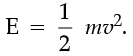The kinetic energy of a spinning object is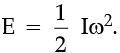Case Based Questions Test: System of Particles & Rotational Motion - Question 10

Flywheel and sewing machine

There is a difference between inertia and moment of inertia of a body. Inertia depends on the mass of the body but the moment of inertia about an axis depends on the mass of the body and the distribution of its mass about the axis. In the following figure, the masses of the two wheels are exactly equal but in the wheel (A) the mass is uniformly distributed and in the wheel (B) most of the mass is situated at the rim. Both the wheels rotate about axis passing through the centre. It is noticed that while the two wheel are set in rotation and left, wheel (B) continues rotating for a longer time.This means that the moment of inertia of wheel (B) is greater than the wheel (B). Also greater is the part of the mass of the body away from the axis of rotation, greater the moment of inertia of the body about the axis. Such a wheel is known as flywheel. Consider a foot operated sewing machine. It has of two wheels – one big and the other small. The wheels are connected by a rope. The bigger wheel acts as flywheel. The rope transfers the motion from this flywheel to the smaller wheel. Smaller wheel works as a pulley and also as a small flywheel.We see even we stop supply of driving force to the bigger wheel it still continues to run for a short time because of its moment of inertia. So, flywheel acts as an energy reservoir by storing and supplying mechanical energy when required. The kinetic energy stored in a flywheel is(where I = moment of inertia and ω = angular velocity).

Which one of the following wheel (having same mass) will have highest moment of inertia about axis passing through the centre?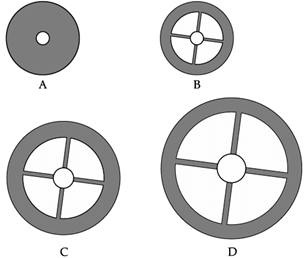Detailed Solution for Case Based Questions Test: System of Particles & Rotational Motion - Question 10
Mass of all the wheels are the same. In wheel D, the mass is distributed furthest from the axis. Since moment of inertia = ∑mr2, hence wheel D will have highest moment of inertia.

## Physics Class 11

130 videos|483 docs|210 tests
Information about Case Based Questions Test: System of Particles & Rotational Motion Page
In this test you can find the Exam questions for Case Based Questions Test: System of Particles & Rotational Motion solved & explained in the simplest way possible. Besides giving Questions and answers for Case Based Questions Test: System of Particles & Rotational Motion, EduRev gives you an ample number of Online tests for practice

## Physics Class 11

130 videos|483 docs|210 tests

### How to Prepare for NEET

Read our guide to prepare for NEET which is created by Toppers & the best Teachers(Scan QR code)